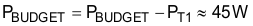SLUS157Q December   1999  – October 2019

PRODUCTION DATA.

1. Features
2. Applications
3. Description
1.     Device Images
4. Revision History
5. Pin Configuration and Functions
6. Specifications
7. Detailed Description
8. Application and Implementation
1. 8.1 Application Information
2. 8.2 Typical Application
1. 8.2.1 Design Requirements
2. 8.2.2 Detailed Design Procedure
3. 8.2.3 Application Curves
9. Power Supply Recommendations
10. 10Layout
11. 11Device and Documentation Support
12. 12Mechanical, Packaging, and Orderable Information

• DW|20
• PW|20
• N|20
• PW|20

#### 8.2.2.2 Preliminary Transformer Calculations (T1)

Transformer turns ratio (a1):

Equation 8.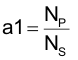The voltage drop across the RDS(on) of the primary side FETs is negligible. We assume a 0.5-V forward voltage drop in the output rectifiers.

Equation 9.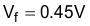Select transformer turns based on 70% duty cycle (DMAX) at minimum specified input voltage. This will give some room for dropout if a PFC front end is used.

Equation 10.Equation 11.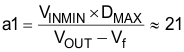Turns ratio rounded to the nearest whole turn.

Equation 12.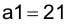Calculated typical duty cycle (DTYP) based on average input voltage.

Equation 13.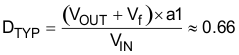Output inductor peak-to-peak ripple current is set to 20% of the output current.

Equation 14.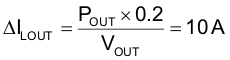Care must be taken in selecting the correct amount of magnetizing inductance (LMAG). The following equations calculate the minimum magnetizing inductance of the primary of the transformer (T1) to ensure the converter operates in current-mode control. As LMAG reduces, the increasing magnetizing current becomes an increasing proportion of the signal at the CS pin. If the magnetizing current increases enough it can swamp out the current sense signal across RCS and the converter will operate increasingly as if it were in voltage mode control rather than current mode.

Equation 15.Figure 19 shows the transformer primary and secondary currents during normal operation.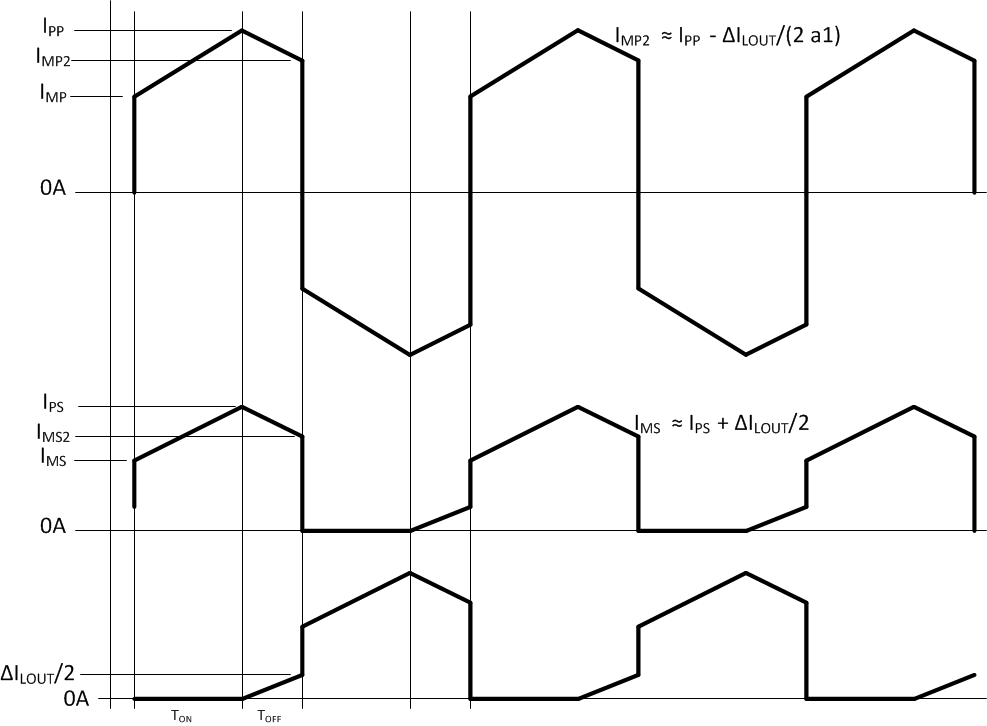Figure 19. T1 Primary and Secondary Currents

Calculate T1 secondary RMS current (ISRMS):

Equation 16.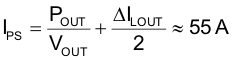Equation 17.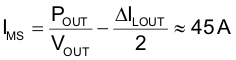Equation 18.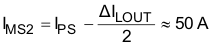Secondary RMS current (ISRMS1) when energy is being delivered to the secondary: (OUTA = OUTD = HI or OUTB = OUTC = HI).

Equation 19.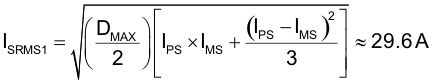Secondary RMS current (ISRMS2) during freewheeling period: (OUTA = OUTC = HI or OUTB = OUTD = HI).

Equation 20.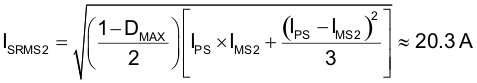Secondary RMS current (ISRMS3) caused by the negative current in the opposing winding during freewheeling period, please refer to Figure 19.

Equation 21.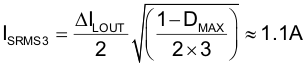Total secondary RMS current (ISRMS):

Equation 22.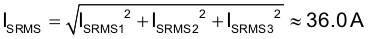Calculate T1 Primary RMS Current (IPRMS):

Equation 23.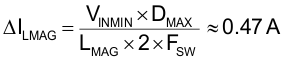Equation 24.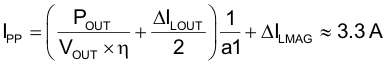Equation 25.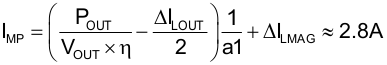Equation 26.Equation 27.T1 Primary RMS (IPRMS1) current when energy is being delivered to the secondary.

Equation 28.T1 Primary RMS (IPRMS2) current when the converter is free wheeling.

Equation 29.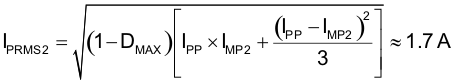Total T1 primary RMS current (IPRMS):

Equation 30.We select a transformer with the following specifications:

Equation 31.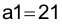Equation 32.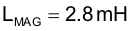Transformer Primary DC resistance:

Equation 33.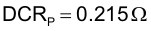Transformer Secondary DC resistance:

Equation 34.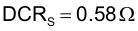Estimated transformer core losses (PT1) are twice the copper loss.

NOTE

This is just an estimate and the total losses may vary based on magnetic design.

Equation 35.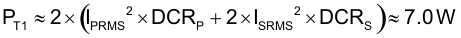Calculate remaining power budget:

Equation 36.